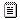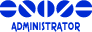Wrote new code? Fixed a bug? Want to discuss technical stuff? Feel free to post it here.

Moderator: Moderators

Message
Author
kali
OpenKore MonkPosts: 474
Joined: 04 Apr 2008, 10:10

Code: Select all

``````		String unpackString = "v2 x20 V4 v x22 x2 v x2 v x2 v2 x2 v2 x2 v x2 v x2 v4 Z24 C6 v x2";
unpack(
true,
unpackString,
charInfo,
qw("header length charID exp zeny jobExp jobLevel hp maxHp sp maxSp jobId hairstyle level headLow headTop headMid hairColor clothesColor name str agi vit intel dex luk slot"));
}``````
Difference with old 006B:
20 bytes skipped from length field to first charID
2 bytes skipped after hp
2 bytes skipped after maxHp
Got your topic trashed by a mod?

Trashing topics is one click, and moving a topic to its proper forum is a lot harder. You expend the least effort in deciding where to post, mods expend the least effort by trashing.

Have a nice day.

kali
OpenKore MonkPosts: 474
Joined: 04 Apr 2008, 10:10

Andy12345, if you're reading this, could you paste here the servers.txt entry for ExperienceRO? So I can test my patches tooGot your topic trashed by a mod?

Trashing topics is one click, and moving a topic to its proper forum is a lot harder. You expend the least effort in deciding where to post, mods expend the least effort by trashing.

Have a nice day.

andy12345
ModeratorsPosts: 174
Joined: 04 Apr 2008, 10:21
Noob?: No

kali wrote:Andy12345, if you're reading this, could you paste here the servers.txt entry for ExperienceRO? So I can test my patches tooSure

Code: Select all

``````[ExpRO]
ip 66.160.197.121
port 6900
version 20
master_version 16
private 1
serverEncoding Western
charBlocksize 132  #this one to experimented with.#
recvpackets recvpackets.txt``````

kali
OpenKore MonkPosts: 474
Joined: 04 Apr 2008, 10:10

Note to self:
charBlockSize must be set to 112
Got your topic trashed by a mod?

Trashing topics is one click, and moving a topic to its proper forum is a lot harder. You expend the least effort in deciding where to post, mods expend the least effort by trashing.

Have a nice day.

kali
OpenKore MonkPosts: 474
Joined: 04 Apr 2008, 10:10

Andy, could you try patching this in as a test?

Code: Select all

``````Index: Receive.pm
===================================================================
@@ -4917,21 +4917,22 @@
for (my \$i = \$args->{RAW_MSG_SIZE} % \$blockSize; \$i < \$args->{RAW_MSG_SIZE}; \$i += \$blockSize) {
#exp display bugfix - chobit andy 20030129
-		\$num = unpack("C1", substr(\$args->{RAW_MSG}, \$i + 104, 1));
+		\$num = unpack("C1", substr(\$args->{RAW_MSG}, \$i + 104+4, 1));
\$chars[\$num] = new Actor::You;
\$chars[\$num]{ID} = \$accountID;
-		\$chars[\$num]{charID} = substr(\$args->{RAW_MSG}, \$i, 4);
+		\$chars[\$num]{charID} = substr(\$args->{RAW_MSG}, \$i-4, 4);
\$chars[\$num]{nameID} = unpack("V", \$chars[\$num]{ID});
+        message \$chars[\$num]{nameID};
\$chars[\$num]{exp} = unpack("V", substr(\$args->{RAW_MSG}, \$i + 4, 4));
-		\$chars[\$num]{zenny} = unpack("V", substr(\$args->{RAW_MSG}, \$i + 8, 4));
-		\$chars[\$num]{exp_job} = unpack("V", substr(\$args->{RAW_MSG}, \$i + 12, 4));
-		\$chars[\$num]{lv_job} = unpack("v", substr(\$args->{RAW_MSG}, \$i + 16, 2));
-		\$chars[\$num]{hp} = unpack("v", substr(\$args->{RAW_MSG}, \$i + 42, 2));
-		\$chars[\$num]{hp_max} = unpack("v", substr(\$args->{RAW_MSG}, \$i + 44, 2));
-		\$chars[\$num]{sp} = unpack("v", substr(\$args->{RAW_MSG}, \$i + 46, 2));
-		\$chars[\$num]{sp_max} = unpack("v", substr(\$args->{RAW_MSG}, \$i + 48, 2));
-		\$chars[\$num]{jobID} = unpack("v", substr(\$args->{RAW_MSG}, \$i + 52, 2));
-		\$chars[\$num]{hair_style} = unpack("v", substr(\$args->{RAW_MSG}, \$i + 54, 2));
+		\$chars[\$num]{zenny} = unpack("V", substr(\$args->{RAW_MSG}, \$i + 8-4, 4));
+		\$chars[\$num]{exp_job} = unpack("V", substr(\$args->{RAW_MSG}, \$i + 12-4, 4));
+		\$chars[\$num]{lv_job} = unpack("v", substr(\$args->{RAW_MSG}, \$i + 16-4, 2));
+		\$chars[\$num]{hp} = unpack("V", substr(\$args->{RAW_MSG}, \$i + 42, 2+2));
+		\$chars[\$num]{hp_max} = unpack("V", substr(\$args->{RAW_MSG}, \$i + 44+2, 2+2));
+		\$chars[\$num]{sp} = unpack("v", substr(\$args->{RAW_MSG}, \$i + 46+4, 2));
+		\$chars[\$num]{sp_max} = unpack("v", substr(\$args->{RAW_MSG}, \$i + 48+4, 2));
+		\$chars[\$num]{jobID} = unpack("v", substr(\$args->{RAW_MSG}, \$i + 52+4, 2));
+		\$chars[\$num]{hair_style} = unpack("v", substr(\$args->{RAW_MSG}, \$i + 54+4, 2));
\$chars[\$num]{lv} = unpack("v", substr(\$args->{RAW_MSG}, \$i + 58, 2));
\$chars[\$num]{headgear}{low} = unpack("v", substr(\$args->{RAW_MSG}, \$i + 62, 2));
\$chars[\$num]{headgear}{top} = unpack("v", substr(\$args->{RAW_MSG}, \$i + 66, 2));``````
Got your topic trashed by a mod?

Trashing topics is one click, and moving a topic to its proper forum is a lot harder. You expend the least effort in deciding where to post, mods expend the least effort by trashing.

Have a nice day.

andy12345
ModeratorsPosts: 174
Joined: 04 Apr 2008, 10:21
Noob?: No

Recv Packets got from the exe
Attachmentsrecvpackets.txt
From Packet extractor,
Experience RO recvpackets.txt

h4rry84
ModeratorsPosts: 234
Joined: 04 Apr 2008, 09:30
Noob?: Yes
Location: My House
Contact:

it's using RagnarokRenewal client ?

here's a sneaky eA code that could help ;p : https://opensvn.csie.org/traccgi/RE/changeset/21kLabMousePosts: 1301
Joined: 24 Apr 2008, 12:02

Don't forget the new packets 07xx that eA supports and we not.

Changes:
https://opensvn.csie.org/traccgi/RE/changeset/21kali
OpenKore MonkPosts: 474
Joined: 04 Apr 2008, 10:10

Does not work yet, it's just a workaround for the first character. I'll code a proper fix later (at least I know my parsing works).
Got your topic trashed by a mod?

Trashing topics is one click, and moving a topic to its proper forum is a lot harder. You expend the least effort in deciding where to post, mods expend the least effort by trashing.

Have a nice day.

kali
OpenKore MonkPosts: 474
Joined: 04 Apr 2008, 10:10

Here is the working patch (specific to ExperienceRO)

Code: Select all

``````Index: src/Network/Receive.pm
===================================================================
@@ -4914,38 +4914,45 @@
}

my \$num;
+	my \$blockSize = 112;
for (my \$i = \$args->{RAW_MSG_SIZE} % \$blockSize; \$i < \$args->{RAW_MSG_SIZE}; \$i += \$blockSize) {
#exp display bugfix - chobit andy 20030129
-		\$num = unpack("C1", substr(\$args->{RAW_MSG}, \$i + 104, 1));
+        my \$unpack_string = "V4 v x22 x2 v x2 v x2 v2 x2 v2 x2 v x2 v x2 v4 Z24 C6 v x2";
+		my (\$cID,\$exp,\$zeny,\$jobExp,\$jobLevel,\$hp,\$maxHp,\$sp,\$maxSp,\$jobId,
+		    \$clothesColor,\$name,\$str,\$agi,\$vit,\$intel,\$dex,\$luk,\$num) =
+		    unpack(\$unpack_string, substr(\$args->{RAW_MSG}, \$i));
+
\$chars[\$num] = new Actor::You;
\$chars[\$num]{ID} = \$accountID;
-		\$chars[\$num]{charID} = substr(\$args->{RAW_MSG}, \$i, 4);
+		\$chars[\$num]{charID} = \$cID;
+		\$chars[\$num]{exp} = \$exp;
+		\$chars[\$num]{zenny} = \$zeny;
+		\$chars[\$num]{exp_job} = \$jobExp;
+		\$chars[\$num]{lv_job} = \$jobLevel;
+		\$chars[\$num]{hp} = \$hp;
+		\$chars[\$num]{hp_max} = \$maxHp;
+		\$chars[\$num]{sp} = \$sp;
+		\$chars[\$num]{sp_max} = \$maxSp;
+		\$chars[\$num]{jobID} = \$jobId;
+		\$chars[\$num]{hair_style} = \$hairstyle;
+		\$chars[\$num]{lv} = \$level;
+		\$chars[\$num]{hair_color} = \$hairColor;
+		\$chars[\$num]{clothes_color} = \$clothesColor;
+		\$chars[\$num]{name} = \$name;
+		\$chars[\$num]{str} = \$str;
+		\$chars[\$num]{agi} = \$agi;
+		\$chars[\$num]{vit} = \$vit;
+		\$chars[\$num]{int} = \$intel;
+		\$chars[\$num]{dex} = \$dex;
+		\$chars[\$num]{luk} = \$luk;
+		\$chars[\$num]{sex} = \$accountSex2;
+
\$chars[\$num]{nameID} = unpack("V", \$chars[\$num]{ID});
-		\$chars[\$num]{exp} = unpack("V", substr(\$args->{RAW_MSG}, \$i + 4, 4));
-		\$chars[\$num]{zenny} = unpack("V", substr(\$args->{RAW_MSG}, \$i + 8, 4));
-		\$chars[\$num]{exp_job} = unpack("V", substr(\$args->{RAW_MSG}, \$i + 12, 4));
-		\$chars[\$num]{lv_job} = unpack("v", substr(\$args->{RAW_MSG}, \$i + 16, 2));
-		\$chars[\$num]{hp} = unpack("v", substr(\$args->{RAW_MSG}, \$i + 42, 2));
-		\$chars[\$num]{hp_max} = unpack("v", substr(\$args->{RAW_MSG}, \$i + 44, 2));
-		\$chars[\$num]{sp} = unpack("v", substr(\$args->{RAW_MSG}, \$i + 46, 2));
-		\$chars[\$num]{sp_max} = unpack("v", substr(\$args->{RAW_MSG}, \$i + 48, 2));
-		\$chars[\$num]{jobID} = unpack("v", substr(\$args->{RAW_MSG}, \$i + 52, 2));
-		\$chars[\$num]{hair_style} = unpack("v", substr(\$args->{RAW_MSG}, \$i + 54, 2));
-		\$chars[\$num]{lv} = unpack("v", substr(\$args->{RAW_MSG}, \$i + 58, 2));
-		\$chars[\$num]{headgear}{low} = unpack("v", substr(\$args->{RAW_MSG}, \$i + 62, 2));
-		\$chars[\$num]{headgear}{top} = unpack("v", substr(\$args->{RAW_MSG}, \$i + 66, 2));
-		\$chars[\$num]{headgear}{mid} = unpack("v", substr(\$args->{RAW_MSG}, \$i + 68, 2));
-		\$chars[\$num]{hair_color} = unpack("v", substr(\$args->{RAW_MSG}, \$i + 70, 2));
-		\$chars[\$num]{clothes_color} = unpack("v", substr(\$args->{RAW_MSG}, \$i + 72, 2));
-		(\$chars[\$num]{name}) = unpack("Z*", substr(\$args->{RAW_MSG}, \$i + 74, 24));
-		\$chars[\$num]{str} = unpack("C1", substr(\$args->{RAW_MSG}, \$i + 98, 1));
-		\$chars[\$num]{agi} = unpack("C1", substr(\$args->{RAW_MSG}, \$i + 99, 1));
-		\$chars[\$num]{vit} = unpack("C1", substr(\$args->{RAW_MSG}, \$i + 100, 1));
-		\$chars[\$num]{int} = unpack("C1", substr(\$args->{RAW_MSG}, \$i + 101, 1));
-		\$chars[\$num]{dex} = unpack("C1", substr(\$args->{RAW_MSG}, \$i + 102, 1));
-		\$chars[\$num]{luk} = unpack("C1", substr(\$args->{RAW_MSG}, \$i + 103, 1));
-		\$chars[\$num]{sex} = \$accountSex2;
\$chars[\$num]{name} = bytesToString(\$chars[\$num]{name});
}
``````
I suggest we create a separate function to specify the unpack string, so that other servertypes can just override that instead of overriding the whole function. So for example (pseudo code):

Code: Select all

``````sub received_characters_unpack_string {
return "unpack string goes here";
}
my (\$charID,\$exp ...) = unpack(\$unpack_string, \$raw_data);
}
``````
Other servertypes will just override sub received_characters_unpack_string if they want to specify a different unpacking algorithm (just like with expro).

AFAIK this is the first time OpenKore will support a server with a unique character info packing. The previous one was a simple change of character block size, this time the change is more drastic. I suggest we adapt our code so it becomes more maintainable n the future in case Gravity decides to change this packing format.

This is also the reason why I am not yet creating a separate servertype for expro - I believe we first need to refactor the function involved before extending it; otherwise we'll be stuck with a tangled mess.

And btw, it seems that whatever I do to change the charBlockSize doesn't work. Changing it in servers.txt or in config.txt doesn't seem to stick (I checked with placing trace messages and logs). Maybe it's just me, but it seems that the function broke somehow, or the specific config.txt entryis not being loaded.
Got your topic trashed by a mod?

Trashing topics is one click, and moving a topic to its proper forum is a lot harder. You expend the least effort in deciding where to post, mods expend the least effort by trashing.

Have a nice day.